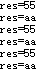``````u8 rece;
u16 dist=0;
u8 relen=0;

int main(void)
{
u8 t=0;
u8 send;
u8 len=sizeof(send);
send=0x55; send=0xaa; send=0x01; send=0x01; send=0x01; send=0x01;
delay_init();
LED_Init();
KEY_Init();
BEEP_Init();
uart_init1(9600);
uart_init2(9600);
TIM3_PWM_Init(7199,9);
TIM_SetCompare2(TIM3,4700);
NVIC_PriorityGroupConfig(NVIC_PriorityGroup_2);
while(1){
for(t=0;t<len;t++){
USART_SendData(USART1,send[t]);
while(USART_GetFlagStatus(USART1,USART_FLAG_TC)!=SET);
}
LED0=0;
LED1=1;
delay_ms(1000);
//printf("\r\ndist=%d   \r\n",dist);
}
}
void USART1_IRQHandler(void){
u8 res;
if(USART_GetITStatus(USART1,USART_IT_RXNE)){
printf("\r\nres=%x   ",res);
}
}
``````

2个回答